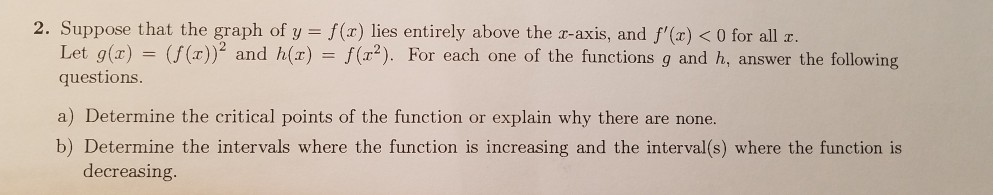# 2. Suppose that the graph of y - f(z) lies entirely above the r-axis and f'(r)

Question
15 viewshelp_outlineImage Transcriptionclose2. Suppose that the graph of y - f(z) lies entirely above the r-axis and f'(r) <0 for all r Let g(x) = (f(x))2 and h(z) = f( 2). For each one of the functions g and h, answer the following questions a) Determine the critical points of the function or explain why there are none. b) Determine the intervals where the function is increasing and the interval(s) where the function is decreasing fullscreen
check_circle

Step 1

(A)

Calculation of critical point of g(x):

we can find derivative

We can see that f(x) is positive and f'(x) is negative

and g'(x) is multiplication of both f(x) and f'(x)

So, g'(x) will become negative because positive*negative =negative

Step 2

Since, it is always negative

So, it can not be equal to 0

So, no critical point exist

No critical point for g(x)

Step 3

Critical point for h(x):

we can find derivative of h(x)

and then we can set derivative to 0

and then we can solve for x

we kno...

### Want to see the full answer?

See Solution

#### Want to see this answer and more?

Solutions are written by subject experts who are available 24/7. Questions are typically answered within 1 hour.*

See Solution
*Response times may vary by subject and question.
Tagged in

### Calculus